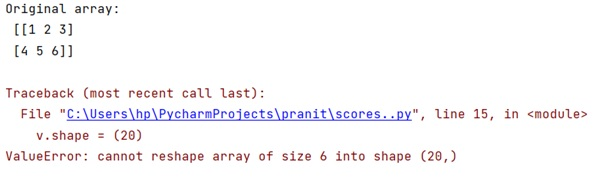# How can I tell if NumPy creates a view or a copy?

Learn, how to identify that if NumPy creates a view or a copy in Python?
Submitted by Pranit Sharma, on January 14, 2023

NumPy is an abbreviated form of Numerical Python. It is used for different types of scientific operations in python. Numpy is a vast library in python which is used for almost every kind of scientific or mathematical operation. It is itself an array which is a collection of various methods and functions for processing the arrays.

## Identifying that if NumPy creates a view or a copy

When we use copy, it makes a new copy of an array and any changes applied to the copied array will not make any impact on the original array.

On the other hand, the view is a representation of the original array where if any changes are made to the view, it will make an impact on the original array or vice-versa.

To identify whether it is a copy or a view, we must understand the working of NumPy reshapes. It is not always possible to change the shape of an array without copying the data. If we want an error to be raised when the data is copied, we should assign the new shape to the shape attribute of the array.

Hence, we can understand that NumPy usually creates a copy when we reshape the NumPy array rather than returning a view.

Let us understand with the help of an example,

## Python code to identify that if NumPy creates a view or a copy

```# Import numpy
import numpy as np

# Creating a numpy array
arr = np.array([[1, 2, 3], [4, 5, 6]])

# Display original array
print("Original array:\n",arr,"\n")

# Transposing array
tr = arr.T

# Create a view and then reshape
v = tr.view()
v.shape = (20)
```

Output:This output explains that a view cannot be made for reshaping the NumPy array to other dimensions.